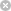RTXI  2.1vector< T > Class Template Reference

`#include <matrix_t.h>`

Inheritance diagram for vector< T >:struct  vrep

## Public Member Functions

vector (int origin, int size)

vector (vector< T > &x)

void PurgeData (void)

~vector (void)

vector< T > & operator= (vector< T > &vec)

vector< T > & operator= (T x)

vector< T > & operator/ (T x)

T * array (void)

T & operator[] (int)

vector< T > & operator* (matrix< T > &m2)

vector (void)

## Protected Attributes

struct vector::vrepp

int Is_Temp

## Friends

class matrix< T >

class rowvec< T >

class colvec< T >

## Detailed Description

### template<class T>class vector< T >

Definition at line 10 of file matrix_t.h.

## Constructor & Destructor Documentation

template<class T >
 vector< T >::vector ( void )
protected

Definition at line 247 of file vector_t.cpp.

template<class T >
 vector< T >::vector ( int origin, int size )

Definition at line 277 of file vector_t.cpp.

template<class T>
 vector< T >::vector ( vector< T > & x )

Definition at line 374 of file vector_t.cpp.

template<class T >
 vector< T >::~vector ( void )

Definition at line 322 of file vector_t.cpp.

## Member Function Documentation

template<class T >
 T * vector< T >::array ( void )

Definition at line 349 of file vector_t.cpp.

template<class T>
 vector< T > & vector< T >::operator* ( matrix< T > & m2 )

Definition at line 536 of file vector_t.cpp.

template<class T>
 vector< T > & vector< T >::operator/ ( T x )

Definition at line 387 of file vector_t.cpp.

template<class T>
 vector< T > & vector< T >::operator= ( vector< T > & vec )

Definition at line 416 of file vector_t.cpp.

template<class T>
 vector< T > & vector< T >::operator= ( T x )

Definition at line 506 of file vector_t.cpp.

template<class T >
 T & vector< T >::operator[] ( int i )

Definition at line 528 of file vector_t.cpp.

template<class T >
 void vector< T >::PurgeData ( void )

Definition at line 260 of file vector_t.cpp.

## Friends And Related Function Documentation

template<class T>
 friend class colvec< T >
friend

Definition at line 50 of file vector_t.h.

template<class T>
 friend class matrix< T >
friend

Definition at line 48 of file vector_t.h.

template<class T>
 friend class rowvec< T >
friend

Definition at line 49 of file vector_t.h.

## Member Data Documentation

template<class T>
 int vector< T >::Is_Temp
protected

Definition at line 31 of file vector_t.h.

template<class T>
 struct vector::vrep * vector< T >::p
protected

The documentation for this class was generated from the following files: